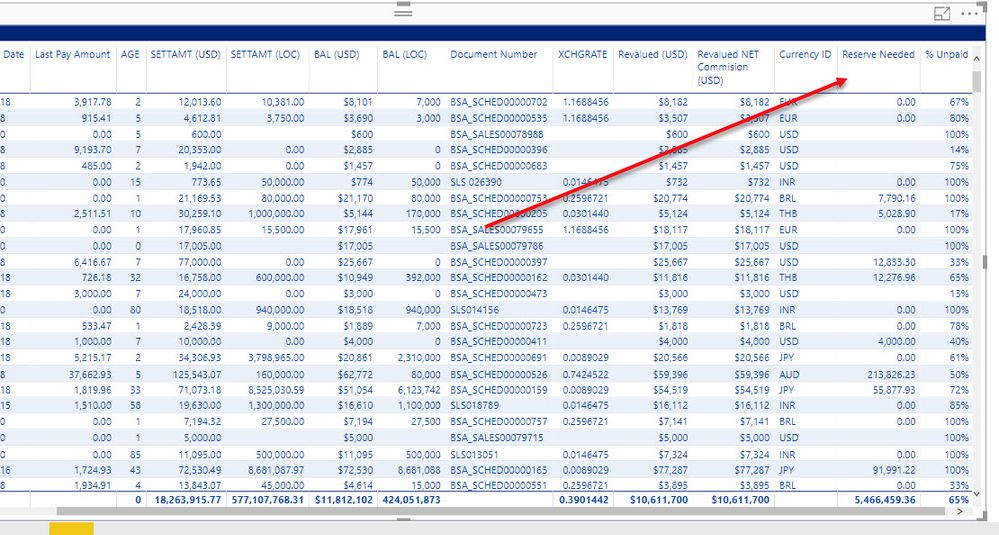cancel
Showing results for
Did you mean:Post Prodigy

## Incorrect Measure Total

Hello,

I am struggling with understanding why measures do not total correctly.

In this report ,  I am trying to perform the following calculation while keeping the filter context on the table.

IF the Invoice Age is equal to or less than 6,  then calculate the reserve * Current Exchange rate otherwise 0.

LTTPEXCHANGE =

SUMX (

VALUES ( '000_BadDebt'[Document Number] ),IF([AGE] <=6,

Then I simply want to show the reserve needed:  IF the document is <=6 then LTTPEXCHANGE else     [Revalued NET Commision (USD)]

[AGE] <=6,

[LTTPEXCHANGE],

[Revalued NET Commision (USD)]

))

However,  the actual row to row calculation works just great, but the actual total does not.

How can I get the calculation to work?

ThankMicrosoft

Hi @lcasey,

What's wrong with the total?

Can you share a sample?

What does "the document is <=6" mean?

Best Regards,

Dale

Community Support Team _ Dale
If this post helps, then please consider Accept it as the solution to help the other members find it more quickly.